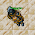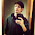## Sunday, July 4, 2021

### TibiaGoals Riddles E10R01

Comment down below!

1.5 + 3 = 8, moving an arrow where it's the number 6, making it number 5 and putting the arrow you removed putting it in the minus sign, making it look like plus.

1.Hello there, Swat Team!

2.My name in tibia is Taekothro

2.Tamarizz - Solidera
You took the bottom left arrow from the 6 to make it a 5, move the arrow to the minus sign to make it a plus.
So 5+3=8

3.6+3=9

Pinguzz

4.By moving the bottom left vertical arrow of the number 8 on top of the - so that it forms a +, and the 8 forms a 9. 6 + 3 = 9.

-Essiee

5.Nehkz
Relania

5+3=8

6.Nehkz
Relania

5+3=8

7.Nehkz
Relania

5+3=8

8.Remove the lower left arrow to make a 5, 5+3 = 8

1.Hello there, fakes!

9.10.Turn the 6 into 5 & the ( - ) into ( + )
5 + 3 = 8

Char: Dyaniixz

11.5+3=8

Mueves el palito inferior izquierdo del 6
By Aurora Ventresca

12.Can you move the arrow from bottom left of number 8 and move that to the minus sign to make it a plus?

13.1.Hello there, Thom!

2.Kanidel Zin

14.6+3=9..... Bebitha consentida

15.6+3=9... Bebitha consentida

16.5+3=8
Vysterion

17.5 + 3 = 8

Char: Marulinha Chatinha

18.6 + 3 = 9

Shizurie

19.6+3=9
Eternal hunger
Antica

20.6+3=9
Eternal hunger
Antica

21.6+3=9
Eternal hunger
Antica

22.6 + 3 = 9.
Mark Argus

23.24.the arrow that is located to the south left of the number "8" is placed on the negative sign to transform it into positive and that the sum of a total of 9

25.Ursi Phin

The arrow that is located to the south left of the number "8" is placed on the negative sign to transform it into positive and that the sum of a total of 9

26.8-3 = 5
You remove an arrow from the 8 (turning a 5) and put it on the 6 (turning an 8)
Name: Tirano Flamel World: Belobra

1.Hello there, Tirano Flamel!

2.Character name: Kottur

27.Nombre : Ursi Phin

Respuesta : the arrow that is located to the south left of the number "8" is placed on the negative sign to transform it into positive and that the sum of a total of 9

Premio : Escojo la runa : Golden Rune Emblem (Desintegrate) y el item para la montura "Four Leaf Clover"

28.An answer to one question that we received: yes, you can indeed take the arrows from the numbers and turn the - into a + etc :)
You can rotate the arrows too!

1.<333333333333

29.Take the bottom left arrow from the 6 to turn the equation into 5+3=8

Xarkost Eilia

30.5+3=8 the arrow pointing up from down left in 6 turning it 5 and putting it on - signal turning it +

31.5+3=8 taking the up arrow from left down on 6 and putting it on - sign

32.5 + 3 = 8 Lord Neganek

33.6+3=9
Mian Stone'arrow

34.I'd move the lower left arrow of the 8 to make the minus a plus so the equation says 6 + 3 = 9. :)
Thanks a lot! - Elyrea

35.1.Hello there, Achiles!

36.Char name:Chilakill Tankmode

37.Respuesta Correctra : 6+3=9 ................ el 8 cambia a 9 y la flecha l colocamos en el signo - a + .................. Zazy Torturador Sombrio

38.Zuali:
https://i.gyazo.com/45cd023d6d0c8b92cba6b21ac9c18a64.png

39.5+3=8

Lovely olka

40.6+3 = 9

Skyelie Hasulhoff <3

41.Name: Matetzera
Answer: 6 + 3 = 9, moving the bottom left arrow of the third number to the 'minus' signal, vertically, to turn '-' into a '+' and '0' into '9'
Reward: I chose a Ultimate Healing rune emblem, and a taming item: Flour Leaf Clover

42.Dolną lewą strzałkę zabrać i zrobić plus z minusa. Otrzymamy wtedy 6 + 3 = 9 Yeka

43.Character: Paollah

Just move the arrow from the number 6 and add to the sign -, turning it into +. Then, 5+3 = 8

44.you can remove the bottom left arrow of the 8 and make the - sign to a plus sign so its 6 + 3 is 9, making the equation correct!

45.5 + 3 = 8
samuray de carro :)

46.aaaaaaaaaaaaaaaaaaaaaaaaaaaaaaaaa

47.move the the first arrow in the left of the 6 to turn it into 5, then put this arrow above the - signal to turn it into +, then u will have 5 + 3 = 8

char: marione the witch

48.you need to change the arrow on the left side of the bottom to turn the 6 into a 5, and place it vertically to change the minus sign to a plus. The answer is 5+3=8

Nickname on Tibia: Karenziinha

49.SE MUEVE LA FLECHA LATERAL IZQUIERDA INFERIOR DEL "6" Y SE COLOCA SOBRE EL SIMBOLO DEL MENOS PARA CONVERTIRLO EN "POSITIVO" (+) HACIENDO QUE LA ECUACION SE CONVIERTA EN 5+3 = 8

NOMBRE DEL PERSONAJE: "ANTHONY RANGEL" SERVIDOR:WINTERA

50.51.Nombre : ANTHONY RANGEL
Respuesta : SE MUEVE LA FLECHA LATERAL IZQUIERDA INFERIOR DEL "6" Y SE COLOCA SOBRE EL SIMBOLO DEL MENOS PARA CONVERTIRLO EN "POSITIVO" (+) HACIENDO QUE LA ECUACION SE CONVIERTA EN "5+3=8"
Premio : Escojo la runa : sudden death y Four Leaf Clover / 5 voodoo doll / collar : Shrunken Head Necklace

1.Hello! This riddle is part of our monthly riddles event, you can find more information about it in here: https://www.tibiagoals.com/p/tibiagoals-riddle-events-rules.html

The prizes such as shrunken head necklace is part of our other event (the riddles for this one will be posted in 4h), you can find more information about it in here: https://www.tibiagoals.com/p/tibiagoals-anniversary-month.html

52.Take the left down arrow from 8 to turn it on 9 and put on - turn it on +

53.Take the left down arrow from 8to turn it on 9 and put on - to form a +

54.55.Easy bottom left arrow of 6 to the - sign and it becomes 5 + 3 = 8

1.Hello there, Ruthlessbob!

56.Take the bottom left arrow from the 6 and move to the - to make it a + which gives you 5+3=8

Toxic Hasulhoff

57.Correct answer: 5 + 3 = 8

by taking an arrow from number 6 and turning it into 5, also arrow turns - into +!

character: pablo reformed

58.remova uma seta do número 6, mude para 5 e mude a operação de subtração para somar

59.remove an arrow from the number 6, change it to 5 and change the subtraction operation to sum

1.Hello there, Dih Negretto!

60.Neo

Moving the bottom left vertical arrow of the 6 to the - sign making it: 5+3=8

61.take 1 arrow from six, become it a 5, add 1 arrow in "-" become it "+", so 5+3=8

62.6+3=9 /Norelli

63.Respuesta : La respuesta move a arrow from number 6 become it a number 5, than get this arrow, become "-" simbol in "+" simbol, so u get equation 5+3=8
Premio : Escojo la runa : Golden Rune Emblem (Paralyze) y music box / 5 voodoo doll / Shrunken Head Necklace

1.Hello! This riddle is part of our monthly riddles event, you can find more information about it in here: https://www.tibiagoals.com/p/tibiagoals-riddle-events-rules.html

The prizes such as shrunken head necklace is part of our other event (the riddles for this one will be posted in 4h), you can find more information about it in here: https://www.tibiagoals.com/p/tibiagoals-anniversary-month.html

64.Remove one arrow from "6" and add it in the "-", which will turn it into a "+". So you will have 5+3 = 8

Char name: Dark Black Magician

65.5 + 3 = 8
Character: Amy Meow

66.6+3=9

character name: Pablo Reformed

67.moving the arrow from the number 8 (lower left) to the sign - to convert to a + and the result would be 6 + 3 = 9

68.69.You can move the bottom left arrow from 6 to make it into a 5 and then move the arrow to - in order to make a +, then the equation becomes right.

1.Hello there, BRBrutus!

70.5+3=8

Pameliin

71.72.73.5+3=8
Ray Jek

74.6 + 3 = 9 , you need to get arrow from 8 :)

75.The answer is take down left arrow from 8 number and put it to - symbol in result we make 6+3=9

76.Forget to add my nickname from game, its Lemori

77.We are happy to welcome so many new participants in our 10th Riddle Event! Please, don't forget to read the rules! Some of you didn't write Tibian nickname, you can find yourself in Riddles/Information in our participant sheet. Or scroll up to check if there is comment under your answer. We won't be able to accept your participation if you won't provide us your real nickname in Tibia.

See you on Wednesday!

78.Take the arrow from 6 turn into a 5 and put the arrow in the - turn into a +
5 + 3 = 8
Sosseguilda - Celebra

79.World: Wintera
Nick: Druid seba

Para resolver este acertijo, debes mover la flecha de izquierda-abajo del número seis hacia el símbolo de resta, convirtiéndolo así en una suma. De esta forma se transforma el seis se transforma en un 5. Entonces nos queda: 5+3=8.
Saludus!

80.Arasaki Nikoledon - Menera

By moving the bottom left corner of 8 to make the '-' sign into a '+' sign.

This changes the equation to 6+3=9